# Program to check Circular Prime Program in Java

In this tutorial you will learn about the Program to check Circular Prime Program in Java and its application with practical example.

In this tutorial, we will learn to create a Java Program to check Circular Prime Program in Java Java

## Prerequisites

Before starting with this tutorial we assume that you are best aware of the following Java programming topics:

• Java Operators.
• Basic Input and Output function in Java.
• Class and Object in Java.
• Basic Java programming.
• If-else statements in Java.
• Function in java.

## Circular Prime Number.

Prime number is called a circular prime if all its cyclic permutations digits,remains a prime number.
Examples:

## Program to check Circular Prime Program in Java

In this program we would find the given number is a Circular Prime number or not , first of all we will take the input from the user and check whether it is Circular Prime number or not. Lets have a look at the code.

## Output

Circular prime number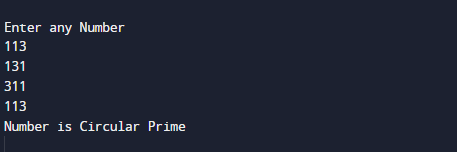Not a Circular prime number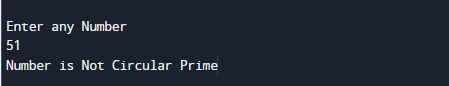In the above program, we have first declared and initialized a set variables required in the program.

• no,num= it will hold entered number.
• temp = it will hold temp value
• sum= for adding digit sum.
• count,ct=  it will hold flag value.
• i,j=  for iteration.

After declaring variables first we take a prime number from user.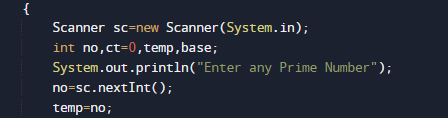then we check all the possible combination of a given number and all are prime number or not.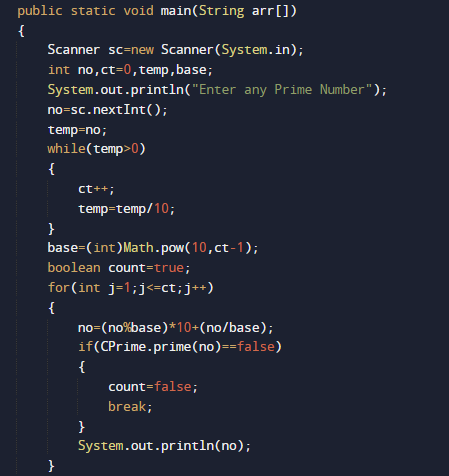Here we created a function prime with in the class Cprime where we checked each and every possible combination of given number and all are prime or not and if they are prime.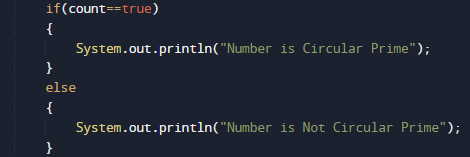A Circular Prime number  is a prime number that remains prime under each every cyclic shifts of its digits.

In this tutorial we have learn about the Program to check Circular Prime Program in Java and its application with practical example. I hope you will like this tutorial.